Next: 5.2 Five Species Phosphorus Up: 5. PROMIS-NT Application Examples Previous: 5. PROMIS-NT Application Examples

# 5.1 Analytical Implantation Simulation

For the development and calibration of diffusion models it is essential to choose proper initial dopant distributions. Since the most common method for introducing electrically charged particles into silicon wafers is ion implantation, suitable initial device descriptions are commonly generated by preceding process simulations. These process simulations include ion implantation simulations which can be classified into two groups based on the underlying modeling approach. The first group is formed by simulators utilizing the Monte Carlo method based on statistical mechanics and atomic target-ion interactions [3,31,6,5]. A second group of ion implantation simulators compute an analytical description of the resulting doping profiles based on statistical moments and distribution functions [22,9,66].

While PROMIS-NT is capable of using the output files of a number of real'' implantation simulators, for simplifying and speeding up the development cycle of new diffusion models it is often preferred to use a once generated input device and to perform the necessary variation of the initial quantity distributions during the setup of the PROMIS-NT diffusion simulation. The quantity initialization mechanism of PROMIS-NT allows for the definition of a simple analytical implantation simulation'' by defining initialization models with built-in distribution functions. The following example utilizes simple two-dimensional Gaussian distribution functions (5.1) - (5.3).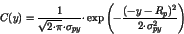(5.1)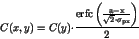(5.2)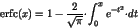(5.3)

The usage of the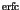function within an MDL input deck requires for the generation of an external MDL function extension library because this function in neither contained in the list of standard MDL functions nor provided by the extensions to the MDL language specification provided by PROMIS-NT. The recommended procedure is described in Appendix A.3.

Example 5.1 lists a possible implementation of the analytical implantation model (5.1) - (5.3). The simulation starts by dynamically loading the requiredfunction and specification of the basic simulator setup Test1InitModel. The assumed test device is depicted in Fig. 5.1. It consists of two segments, the silicon segment targeted by the analytical implantation simulation is located at negative values of the vertical PIF coordinate axis y, and the covering resist segment starting at the lateral coordinate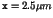. Suiting to the orthogonal structure of this device and the expected results an orthogonal simulation grid is chosen. Since in this example only the initial distribution within the silicon segment is of interest, the simulation time is set to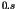and no quantity distributions are computed within the resist segment.

The actual implantation is performed by utilizing the quantity initialization mechanism in the evaluate method of the P_Implant_Init Model class. Local Parameters are used to fit the two-dimensional Gaussian distribution function (5.2) to the results of an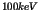phosphorus implant . The resulting phosphorus distribution is depicted in Fig. 5.2.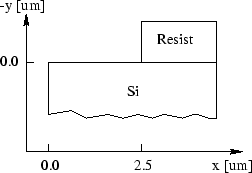Instance PromisNTSetup = Test1InitModel;
NewModel Test1InitModel : PromisNTSetupModel {
evaluate {
:inputPBF  = "inputgrid.pbf";
:outputPBF = "result.pbf";
:endTime   = "0. s";
:deviceSetup           = "P_Pair_Diff_DeviceSetup";
:diffusionModel["Si"]  = "Promis";
}
}

NewModel P_Pair_Diff_DeviceSetup : DeviceSetupModel {
evaluate {
:segmentPreProcessingSetup["Si"] = "SiSegmentPreprocessingSetup";
}
}

NewModel SiSegmentPreprocessingSetup : SegmentPreprocessingSetupModel {
evaluate {
:quantityInitialization["P"] = "P_Implant_Init";
}
}

NewModel SiSegmentPost : SegmentPostprocessingSetupModel {
evaluate {
:quantityPostprocessing["P"] = "No_Post";
}
}

NewModel P_Implant_Init : QuantityInitializationModel {
Local {
// Parameters for a P implant at 100 keV
Parameter<double> spx = 0.055;
Parameter<double> spy = 0.045;
Parameter<double> Rp  = 0.11;
Parameter<double> pi  = acos(-1.0);
Parameter<double> a   = 2.5;
}

evaluate {
:Cown =  1.0e16 / ( sqrt(2.0*pi)*spy );
:Cown *= exp ( - (-:y - Rp)*(-:y - Rp)/(2.0*spy*spy));
:Cown *= erfc( - (a-:x)/(sqrt(2.0)*spx))/2.0;
:Cown += 1.0e5;
}
}

NewModel No_Post : QuantityPostprocessingModel {}

Example 5.1: PROMIS-NT input deck for the analytical implantation simulation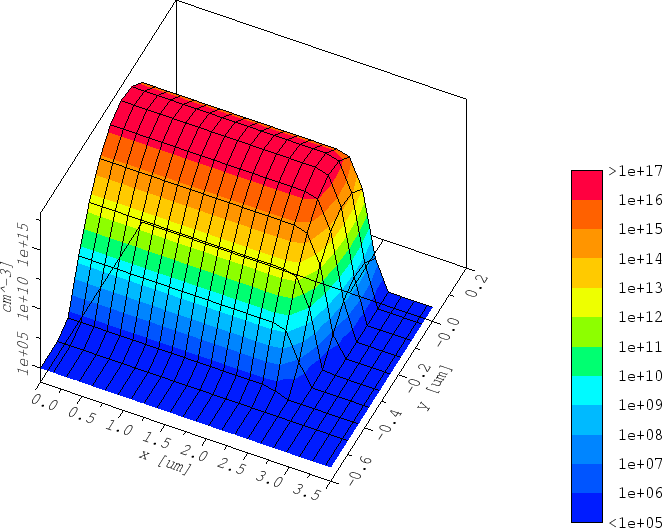Next: 5.2 Five Species Phosphorus Up: 5. PROMIS-NT Application Examples Previous: 5. PROMIS-NT Application Examples
Robert Mlekus
1999-11-14